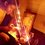# Derivative of peculiar function II

Welcome to the part II of derivative of peculiar functions , well .. we will be discussing only one function. It is a good one. Inspired by Michael Huang and Pi Han Goh . Let's dive right into it!

$\large f^{(0)}(x)_{(n)} = x^{x^{x^{x \hspace{2mm} \cdots \small\text{ upto n ' } x \text{ '} }}}$

$\large f^{(\text{Represents no. of times the func. is diff. w.r.t } x)}(x)_{(\text{Represents number of } x \text{ in the tower })} \hspace{1mm}$ $\hspace{1mm} n \in N$

Now let's take natural log on both sides .

$\ln{(f^{(0)}(x)_{(n)})} = f^{(0)}(x)_{(n-1)} \ln{x}$

$\dfrac{1}{f^{(0)}(x)_{(n)}} \cdot f^{(1)}(x)_{(n)} = f^{(1)}(x)_{(n-1)} \cdot \ln{x} + \dfrac{1}{x} \cdot f^{(0)}(x)_{(n-1)}$

\begin{aligned} f^{(1)}(x)_{(n)} & = f^{(0)}(x)_{(n)} \left(f^{(1)}(x)_{(n-1)} \ln{x} + \dfrac{1}{x}\cdot f^{(0)}(x)_{(n-1)}\right) \\ f^{(1)}(x)_{(n-1)} & = f^{(0)}(x)_{(n-1)} \left( f^{(1)}(x)_{(n-2)} \ln{x} + \dfrac{1}{x}\cdot f^{(0)}(x)_{(n-2)}\right) \hspace{5mm} \times f^{(0)}(x)_{(n)} (\ln{x}) \\ f^{(1)}(x)_{(n-2)} & = f^{(0)}(x)_{(n-2)} \left( f^{(1)}(x)_{(n-3)} \ln{x} + \dfrac{1}{x}\cdot f^{(0)}(x)_{(n-3)}\right) \hspace{5mm} \times f^{(0)}(x)_{(n)}\cdot f^{(0)}(x)_{(n-1)} (\ln{x})^2 \\ \vdots \\ f^{(1)}(x)_{(3)} & = f^{(0)}(x)_{(3)} \left( f^{(1)}(x)_{(2)} \ln{x} + \dfrac{1}{x}\cdot f^{(0)}(x)_{(2)}\right) \hspace{4mm} \times \left( f^{(0)}(x)_{(n)} f^{(0)}(x)_{(n-1)} \cdots f^{(0)}(x)_{(4)} \right) (\ln{x})^{n-3} \\ f^{(1)}(x)_{(2)} & = f^{(0)}(x)_{(2)} \left( f^{(1)}(x)_{(1)} \ln{x} + \dfrac{1}{x}\cdot f^{(0)}(x)_{(1)}\right) \hspace{4mm} \times \left( f^{(0)}(x)_{(n)} f^{(0)}(x)_{(n-1)} \cdots f^{(0)}(x)_{(4)} f^{(0)}(x)_{(3)} \right) (\ln{x})^{n-2} \\ f^{(1)}(x)_{(1)} & = f^{(0)}(x)_{(1)} \left( f^{(1)}(x)_{(0)} \ln{x} + \dfrac{1}{x}\cdot f^{(0)}(x)_{(0)}\right) \hspace{4mm} \times \left( f^{(0)}(x)_{(n)} f^{(0)}(x)_{(n-1)} \cdots f^{(0)}(x)_{(4)} f^{(0)}(x)_{(3)} f^{(0)}(x)_{(2)} \right) (\ln{x})^{n-1} \end{aligned}

\begin{aligned} f^{(1)}(x)_{(n)} & = \dfrac{1}{x}f^{(0)}(x)_{(n)}\cdot f^{(0)}(x)_{(n-1)}+ \dfrac{1}{x}f^{(0)}(x)_{(n)}\cdot f^{(0)}(x)_{(n-1)}\cdot f^{(0)}(x)_{(n-2)} (\ln{x})+ \\ & +\dfrac{1}{x}f^{(0)}(x)_{(n)}\cdot f^{(0)}(x)_{(n-1)}\cdot f^{(0)}(x)_{(n-2)} \cdot f^{(0)}(x)_{(n-3)} (\ln{x})^2 \\ \vdots \\& +\dfrac{1}{x}\left( f^{(0)}(x)_{(n)} f^{(0)}(x)_{(n-1)} \cdots f^{(0)}(x)_{(4)} f^{(0)}(x)_{(3)} f^{(0)}(x)_{(2)} \right) (\ln{x})^{n-3} \\ & +\dfrac{1}{x}\left( f^{(0)}(x)_{(n)} f^{(0)}(x)_{(n-1)} \cdots f^{(0)}(x)_{(4)} f^{(0)}(x)_{(3)} f^{(0)}(x)_{(2)}f^{(0)}(x)_{(1)} \right) (\ln{x})^{n-2} \\ & +\dfrac{1}{x}\left( f^{(0)}(x)_{(n)} f^{(0)}(x)_{(n-1)} \cdots f^{(0)}(x)_{(4)} f^{(0)}(x)_{(3)} f^{(0)}(x)_{(2)} f^{(0)}(x)_{(1)}f^{(0)}(x)_{(0)} \right) (\ln{x})^{n-1} \end{aligned}

$\large \boxed{ f^{(1)}(x)_{(n)} = \dfrac{1}{x}f^{(0)}(x)_{(n)}\cdot f^{(0)}(x)_{(n-1)}\left(1+ \displaystyle\sum_{r=1}^{n-1}\left( \prod_{i=n-r-1}^{n-2} f^{(0)}(x)_{(i)} \right) ( \ln{x} )^{r} \right) }$

Let's Check for $n = 2$

$f^{(1)}(x)_{(2)} = \dfrac{1}{x}f^{(0)}(x)_{(2)}\cdot f^{(0)}(x)_{(1)}\left(1+ \displaystyle\sum_{r=1}^{1}\left( \prod_{i=1-r}^{0} f^{(0)}(x)_{(i)} \right) ( \ln{x} )^{r} \right)$

$\dfrac{d\left(x^x\right)}{dx} = \dfrac{1}{x} \cdot x^x \cdot x \left( 1 + \ln{x} \right) = x^x(1+\ln{x} )$

For $n = 3$

$\dfrac{d\left(x^{x^x}\right)}{dx} = \dfrac{1}{x} \cdot x^{x^x} \cdot x^x \left( 1 + x\ln{x}+ x(\ln{x})^2 \right)$

Master challenge : Can you do it for $f^{(m)}(x)_{(n)}$, where $m$ is a natural number?Note by Sabhrant Sachan
4 years, 5 months ago

This discussion board is a place to discuss our Daily Challenges and the math and science related to those challenges. Explanations are more than just a solution — they should explain the steps and thinking strategies that you used to obtain the solution. Comments should further the discussion of math and science.

When posting on Brilliant:

• Use the emojis to react to an explanation, whether you're congratulating a job well done , or just really confused .
• Ask specific questions about the challenge or the steps in somebody's explanation. Well-posed questions can add a lot to the discussion, but posting "I don't understand!" doesn't help anyone.
• Try to contribute something new to the discussion, whether it is an extension, generalization or other idea related to the challenge.

MarkdownAppears as
*italics* or _italics_ italics
**bold** or __bold__ bold
- bulleted- list
• bulleted
• list
1. numbered2. list
1. numbered
2. list
Note: you must add a full line of space before and after lists for them to show up correctly
paragraph 1paragraph 2

paragraph 1

paragraph 2

[example link](https://brilliant.org)example link
> This is a quote
This is a quote
    # I indented these lines
# 4 spaces, and now they show
# up as a code block.

print "hello world"
# I indented these lines
# 4 spaces, and now they show
# up as a code block.

print "hello world"
MathAppears as
Remember to wrap math in $$ ... $$ or $ ... $ to ensure proper formatting.
2 \times 3 $2 \times 3$
2^{34} $2^{34}$
a_{i-1} $a_{i-1}$
\frac{2}{3} $\frac{2}{3}$
\sqrt{2} $\sqrt{2}$
\sum_{i=1}^3 $\sum_{i=1}^3$
\sin \theta $\sin \theta$
\boxed{123} $\boxed{123}$